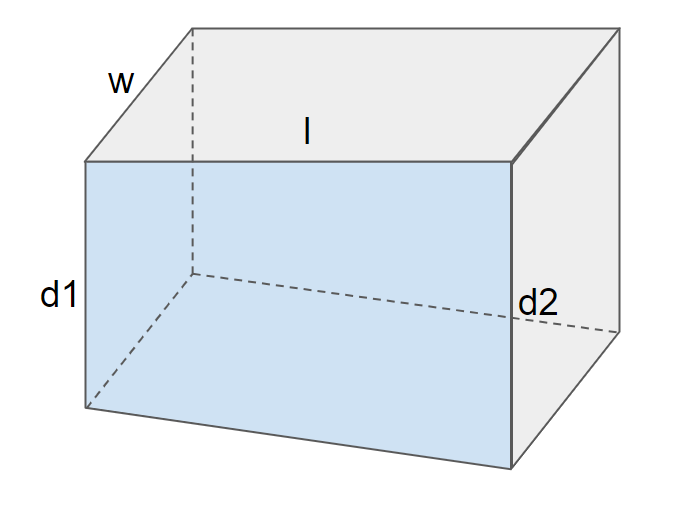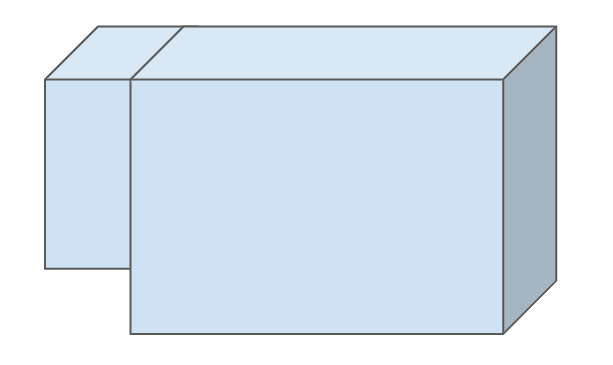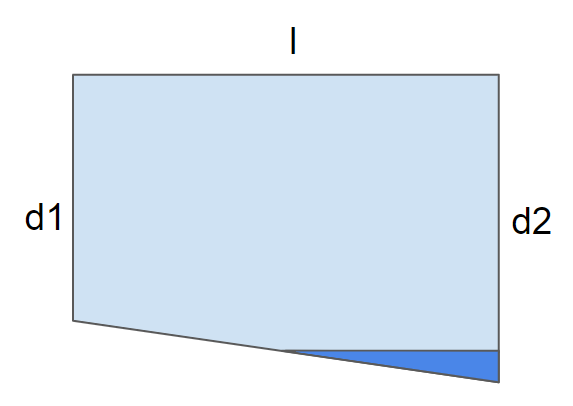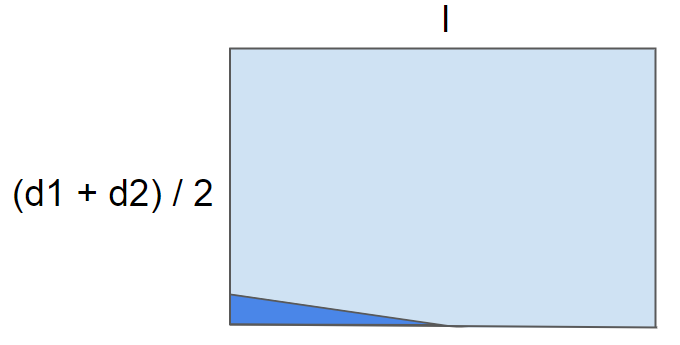# Pool Calculator

Created by Piotr Małek, Mateusz Mucha and Hanna Pamuła, PhD candidate
Reviewed by Bogna Szyk and Jack Bowater
Last updated: Apr 12, 2022

The pool calculator tells you how much water you will need to fill pools of various sizes and depths. 🏊 This is useful information that will help you to better estimate the cost of the water, as well as the amount of time you need to go from an empty pool to floating around with a cool drink in your hand. If you're wondering how many gallons your pool has, give this tool a try!

Please note that the pool volume calculator assumes that the depth of the pool changes evenly and gradually from one end to the other, as is generally the case. If this does not apply to your pool size, figures computed by the pool calculator are still be in the right ballpark.

## How does the pool volume calculator work?

The pool water calculator is a tool similar to the volume calculator; it also uses also the formulas from the square footage calculator.Finding the volume of a pool is a simple matter of getting the area of the surface of the pool and then multiplying that by the average depth.

• Start by entering the pool size (length and width).
• Then add its depth at the two endpoints.
• If there is both a shallow and a deep end, enter the shallow depth in the first field and the deepest point in the second. Otherwise, enter two identical values.
• If the depth of your pool changes drastically instead of gradually, you may want to consider dividing it in half, with a separate pool calculator measurement for each part (as in the image on the right).You could go one step further and, knowing the density of water (which is approximately 1 kilogram per liter), work out how much the water in such a pool would weight.

## Formulas behind pool calculator

So you no longer need to worry "how many gallons is my pool?", simply apply one of the formulas:

1. Rectangular / square pool

volume = length * width * (depth1 + depth2) / 2

The first two agents is, of course, the formula for the area of the rectangle.

1. Oval / circle pool

volume = (π * length * width / 4) * (depth1 + depth2) / 2

The first part of the equation comes from a standard area of an ellipsis formula: A = π * a * b, where a, b are the distances between the center and the ellipse's vertex, lying on the horizontal and vertical axes. In our case, we have the length and width values that are the doubled distances a and b, so in the final formula, we need to divide the surface area of an oval by 4.You are wondering why we average the depths (depth1 + depth2) / 2? Have a look at the pictures:

1. Here you have the standard rectangular pool. l stands for the length of the pool, w for width, d1 is depth on one end of the pool and d2 is the depth on the other end.1. Let's take the right trapezoidal side of the pool. "Cut" the triangle, with one apex in the midpoint of the side, as in the picture.

1. Flip and move it to the other side to form a rectangle. The edge of the new shape is the average of two sides, so

(d1 + d2) / 2.The area of such a rectangle is then length * (depth1 + depth2) / 2. Multiply it by the third dimension - the width - and you'll get the final formula for rectangular pool volume:

• volume = length * width * (depth1 + depth2) / 2

## How many gallons is my pool?

Let's calculate it with this pool volume calculator!

1. Choose the shape of your pool. You can select between two types - rectangular/square pool or oval/circle one. Our example is with a standard rectangular pool, so leave the default value.

2. Enter the dimensions of the swimming pool. Let's say that the pool is 12 ft wide and 50 ft long. Depth is gradually changing from 4 to 6 feet.

3. The pool calculator shows the volume of water needed to fill up the pool. In our case, it's 22,441.57 US gallons.

Our tool is sometimes called the pool size calculator or pool gallon calculator because it works with any units, not just with metric. If we didn't auto-detect your preferred units, click on meters to change it to gallons.

If your pool doesn't vary in depth, but is some kind of a fancy shape like bow front or cylinder, check out this aquarium calculator - it has some nice options for different 3D shapes. If the pool of your dreams comes in some custom or eccentric shape, check out the manufacturer's website.

Advanced mode:

1. If you know the price of water in your area, you can calculate the cost of filling up your pool. Click on the Advanced mode button, and an additional part will appear. The pool gallon calculator takes the result of the previous section and multiplies it by the applicable rates of your local water supply. And thats it, all you need to do to find out how much beating the heat will cost!

Let's take the average cost of the water in the US, which is $1.50 per 1000 gallons. For our exemplary pool, the cost of water is$33.66. Not so bad, huh?

This calculator finds the total volume of your pool - in reality, you never fill the pool all the way to the brim. Also, you may want to have a look at our other tool associated with submerged objects and their volume and weight - the buoyancy calculator.

## Olympic swimming pool volume

To calculate the Olympic swimming pool volume, you need to know its dimensions. The length of the pool is 50 m, width 25 m, and the depth varies: 2.0 m is the minimum, but 3.0 m is the recommended depth. If you're not familiar with those metric units, check out this length converter to convert easily the pool size to US or imperial units.

Olympic-size swimming pool contains 2,500,000 L of water, assuming a nominal depth of 2 m. It's around 550,000 imp gal and 660,000 US gal - if you don't believe us, you can check it with our volume converter tool, where you'll find other volume units as well. Try to calculate the Olympic swimming pool volume for a recommended depth of 3 meters - or use the pool calculator to get the answer in the blink of an eye.

Have you ever wondered how many tea- or tablespoons of water can fit into the Olympic swimming pool? We haven't either. But now you can check it! Awesome, isn't it?

If you're thinking about constructing🔨 your own pool or pondpond in the backyard, you may want to calculate the amount of paint or tiles needed, and also the amount of pool salt and chlorine to be added.

The pool may also bring holidays to your mind - when laying on the beach🌴 or by the pool, don't forget about putting sunscreen on! Make sure you know how long you can safely stay in the sun and which SPF factor to use - have a look at our amazing sunbathing calculator👙 to find the answers.

If you associate the word pool with sports and fitness🏊, you may want to check how many calories you'll burn during a swim - give this calories burned calculator a go! Also, you may find the tools for assessing your speed or pace quite useful.

## FAQ

### How to calculate the water I need for my pool?

To find the water you need for your pool:

1. Measure the length and width of your pool.
2. Measure the depth of the pool from where you'd like the waterline to be. If your pool's depth varies, take the average depth of both ends.
3. Ensure length, width, and depth are all in the same units.
4. Multiply the three measurements together to get your pool volume.

### How much chlorine do I need for my pool?

To find the amount of chloride your pool needs:

1. Determine the volume of your pool.
2. Refer to the chlorine manufacturer and find the ratio of water/chlorine you need to use. You can also consider using 52-104 oz of liquid chlorine (1.5 - 3.1 liters ) per 10,000 gallons of water (38 cubic meters). The equivalent ratio is 8.15 liters per 100 cubic meters.
3. Multiply your pool volume by the chosen ratio, keeping both units of volume the same, and you will get your chlorine requirement.

### How many gallons do I need for my pool?

To find the amount of gallons you need to fill your pool:

1. Measure the length and width of your pool in feet. You also need to know the average depth in feet.
2. Determine the volume by multiplying all three measurements together. The result will be in cubic feet.
3. Multiply the result by 7.5 to convert the volume into US gallons.

### How much it cost to fill my pool?

To find the cost to fill you pool:

1. Measure the length, width, and average depth of your pool.
2. Multiply all three values together to find the volume.
3. Find out the cost of water per unit volume from with your local water provider.
4. Multiply the total water requirement per unit price to get the total cost.
Piotr Małek, Mateusz Mucha and Hanna Pamuła, PhD candidate
Pool shape
rectangle
Dimensions
Width
ft
Length
ft
Depth 1
ft
Depth 2
ft
Volume
Pool volume
US gal
People also viewed…

### Car heat

The hot car calculator shows you how fast the interior of a car heats up during a summers day.

### Plate weight

This plate weight calculator will help you determine the weight of a plate-shaped material of any area, thickness, and density.

### Sunbathing

Do you always remember to put on sunscreen before going outside? Are you sure that you use enough? The Sunbathing Calculator ☀ will tell you when's the time to go back under an umbrella not to suffer from a sunburn!

### Tank volume

Learn how to find the volume of different containers with this tank volume calculator.